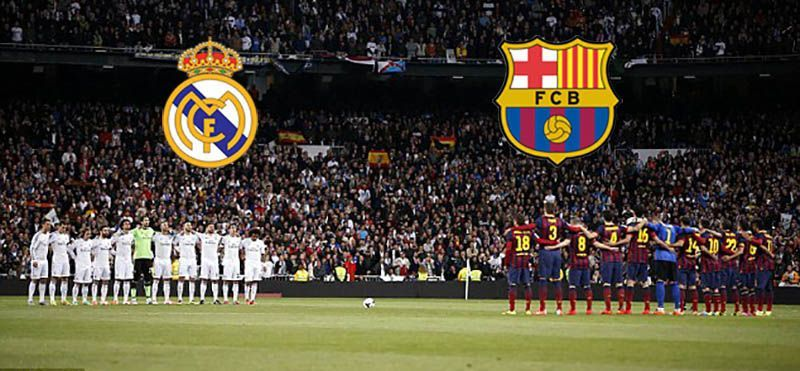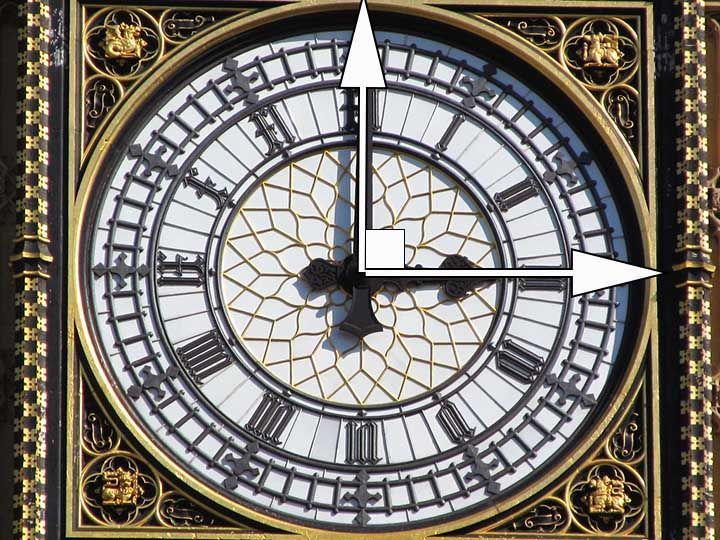# Lenders and Borrowers

Borrowers borrow money when they need it. They borrow it from lenders, who charge an interest on it.

# Principal, Amount, Interest

The sum of money borrowed is called the Principal, the sum paid back is called the Amount. And the difference between the Amount and Principal is the interest.

Amount = Principal + Interest

Example: If I borrow a Principal of Rs. 100 and pay back and Amount of Rs. 115, the Interest is Rs. 15.

Amount = Principal + Interest

Principal = Amount - Interest

Interest = Amount - Principal

# Rate

If you give your friend a 100 rupees and charge him 15 rupees as interest after 1 year, the rate will be 15 per-cent.

We learned in a previous episode, that 'per cent' is nothing but 'for every hundred'.

For 100 rupees lent, you are asking for 15 rupees extra. Which means the interest is 15 Rs. for every 100 rupees. That is, 15 'percent'.

# Interest depends on Time!

The value of interest also depends on the time period for which the Principal is borrowed.

If Rs. 1000 are borrowed at a rate of 10% per annum, the interest charged is Rs. 100.

If the same amount is borrowed at the same rate for 2 years, the interest becomes double, that is, Rs. 200.

# Access All Content

## More from Grade 7 Math# Sets

Sets are around us, everywhere. Learn how they are formed, named, and represented.# Types of Lines

All you need to know about lines, parallel, perpendicular and intersecting lines. Along with all the examples from the world around you.# Types of Angles

Learn about the different types of angles acute, right, obtuse and more through exciting facts from the Wonders of the World.